# Photo resistor devices its working and applications.

9. Apr 2015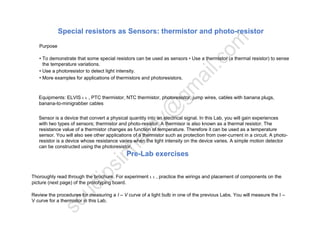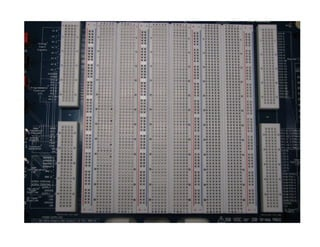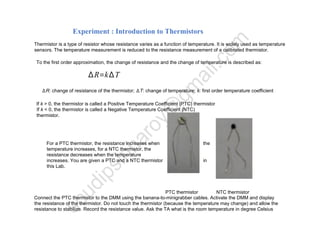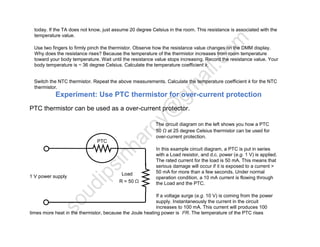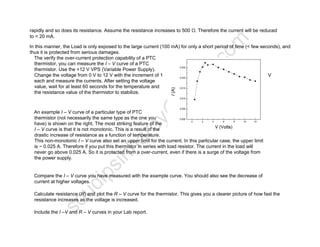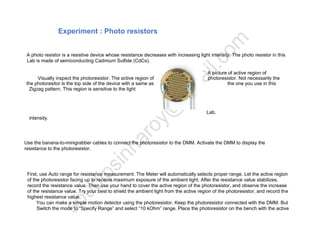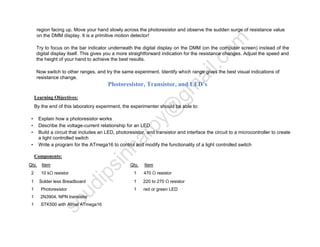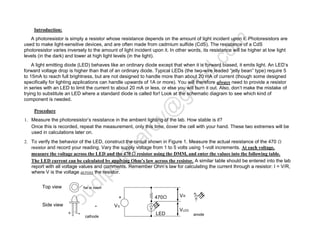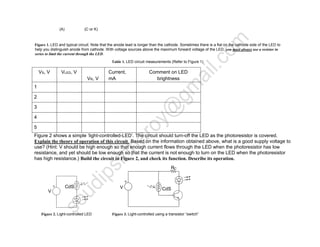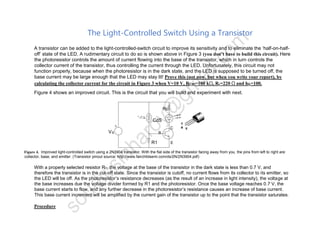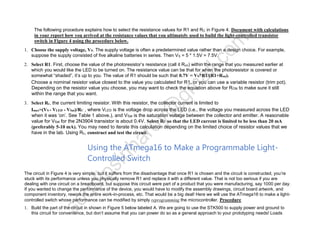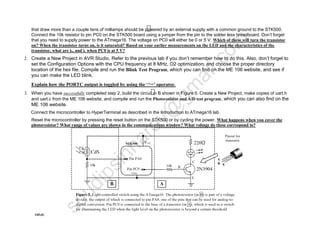1 von 14

### Photo resistor devices its working and applications.

• 1. Special resistors as Sensors: thermistor and photo-resistor Purpose • To demonstrate that some special resistors can be used as sensors • Use a thermistor (a thermal resistor) to sense the temperature variations. • Use a photoresistor to detect light intensity. • More examples for applications of thermistors and photoresistors. Equipments: ELVIS ι ι , PTC thermistor, NTC thermistor, photoresistor, jump wires, cables with banana plugs, banana-to-minigrabber cables Sensor is a device that convert a physical quantity into an electrical signal. In this Lab, you will gain experiences with two types of sensors; thermistor and photo-resistor. A thermisor is also known as a thermal resistor. The resistance value of a thermistor changes as function of temperature. Therefore it can be used as a temperature sensor. You will also see other applications of a thermistor such as protection from over-current in a circuit. A photo- resistor is a device whose resistance varies when the light intensity on the device varies. A simple motion detector can be constructed using the photoresistor. Pre-Lab exercises Thoroughly read through the brochure. For experiment ι ι , practice the wirings and placement of components on the picture (next page) of the prototyping board. Review the procedures for measuring a I – V curve of a light bulb in one of the previous Labs. You will measure the I – V curve for a thermistor in this Lab.
• 2. You have measured I – V curve of a light bulb. In this Lab, you will measure the I – V curve of a PTC thermistor. From what you have read in the brochure. Compare the similarities and differences between a light bulb and a PTC thermistor.
• 4. Experiment : Introduction to Thermistors Thermistor is a type of resistor whose resistance varies as a function of temperature. It is widely used as temperature sensors. The temperature measurement is reduced to the resistance measurement of a calibrated thermistor. To the first order approximation, the change of resistance and the change of temperature is described as: ∆R=k∆T ∆R: change of resistance of the thermistor; ∆T: change of temperature; k: first order temperature coefficient If k > 0, the thermistor is called a Positive Temperature Coefficient (PTC) thermistor If k < 0, the thermistor is called a Negative Temperature Coefficient (NTC) thermistor. For a PTC thermistor, the resistance increases when the temperature increases, for a NTC thermistor, the resistance decreases when the temperature increases. You are given a PTC and a NTC thermistor in this Lab. PTC thermistor NTC thermistor Connect the PTC thermistor to the DMM using the banana-to-minigrabber cables. Activate the DMM and display the resistance of the thermistor. Do not touch the thermistor (because the temperature may change) and allow the resistance to stabilize. Record the resistance value. Ask the TA what is the room temperature in degree Celsius
• 5. today. If the TA does not know, just assume 20 degree Celsius in the room. This resistance is associated with the temperature value. Use two fingers to firmly pinch the thermistor. Observe how the resistance value changes on the DMM display. Why does the resistance rises? Because the temperature of the thermistor increases from room temperature toward your body temperature. Wait until the resistance value stops increasing. Record the resistance value. Your body temperature is ~ 36 degree Celsius. Calculate the temperature coefficient k. Switch the NTC thermistor. Repeat the above measurements. Calculate the temperature coefficient k for the NTC thermistor. Experiment: Use PTC thermistor for over-current protection PTC thermistor can be used as a over-current protector. The circuit diagram on the left shows you how a PTC 50 Ω at 25 degree Celsius thermistor can be used for over-current protection. In this example circuit diagram, a PTC is put in series with a Load resistor, and d.c. power (e.g. 1 V) is applied. The rated current for the load is 50 mA. This means that serious damage will occur if it is exposed to a current > 50 mA for more than a few seconds. Under normal operation condition, a 10 mA current is flowing through the Load and the PTC. If a voltage surge (e.g. 10 V) is coming from the power supply. Instantaneously the current in the circuit increases to 100 mA. This current will produces 100 times more heat in the thermistor, because the Joule heating power is I2R. The temperature of the PTC rises PTC 1 V power supply Load R = 50 Ω
• 6. rapidly and so does its resistance. Assume the resistance increases to 500 Ω. Therefore the current will be reduced to < 20 mA. In this manner, the Load is only exposed to the large current (100 mA) for only a short period of time (< few seconds), and thus it is protected from serious damages. The verify the over-current protection capability of a PTC thermistor, you can measure the I – V curve of a PTC thermistor. Use the +12 V VPS (Variable Power Supply). Change the voltage from 0 V to 12 V with the increment of 1 V each and measure the currents. After setting the voltage value, wait for at least 60 seconds for the temperature and the resistance value of the thermistor to stabilize. An example I – V curve of a particular type of PTC thermistor (not necessarily the same type as the one you have) is shown on the right. The most striking feature of the I – V curve is that it is not monotonic. This is a result of the drastic increase of resistance as a function of temperature. This non-monotonic I – V curve also set an upper limit for the current. In this particular case, the upper limit is ~ 0.025 A. Therefore if you put this thermistor in series with load resistor. The current in the load will never go above 0.025 A. So it is protected from a over-current, even if there is a surge of the voltage from the power supply. Compare the I – V curve you have measured with the example curve. You should also see the decrease of current at higher voltages. Calculate resistance (R) and plot the R – V curve for the thermistor. This gives you a clearer picture of how fast the resistance increases as the voltage is increased. Include the I –V and R – V curves in your Lab report.
• 7. Experiment : Photo resistors A photo resistor is a resistive device whose resistance decreases with increasing light intensity. The photo resistor in this Lab is made of semiconducting Cadmium Sulfide (CdCs). A picture of active region of Visually inspect the photoresistor. The active region of photoresistor. Not necessarily the the photoresitor is the top side of the device with a same as the one you use in this Zigzag pattern. This region is sensitive to the light Lab. intensity. Use the banana-to-minigrabber cables to connect the photoresistor to the DMM. Activate the DMM to display the resistance to the photoresistor. First, use Auto range for resistance measurement. The Meter will automatically selects proper range. Let the active region of the photoresistor facing up to receive maximum exposure of the ambient light. After the resistance value stabilizes, record the resistance value. Then use your hand to cover the active region of the photoresistor, and observe the increase of the resistance value. Try your best to shield the ambient light from the active region of the photoresistor, and record the highest resistance value. You can make a simple motion detector using the photoresistor. Keep the photoresistor connected with the DMM. But Switch the mode to “Specify Range” and select “10 kOhm” range. Place the photoresistor on the bench with the active
• 8. region facing up. Move your hand slowly across the photoresistor and observe the sudden surge of resistance value on the DMM display. It is a primitive motion detector! Try to focus on the bar indicator underneath the digital display on the DMM (on the computer screen) instead of the digital display itself. This gives you a more straightforward indication for the resistance changes. Adjust the speed and the height of your hand to achieve the best results. Now switch to other ranges, and try the same experiment. Identify which range gives the best visual indications of resistance change. Photoresistor, Transistor, and LED’s Learning Objectives: By the end of this laboratory experiment, the experimenter should be able to: • Explain how a photoresistor works • Describe the voltage-current relationship for an LED • Build a circuit that includes an LED, photoresistor, and transistor and interface the circuit to a microcontroller to create a light controlled switch • Write a program for the ATmega16 to control and modify the functionality of a light controlled switch Components: Qty. Item Qty. Item 2 10 kΩ resistor 1 470 Ω resistor 1 Solder less Breadboard 1 220 to 270 Ω resistor 1 Photoresistor 1 red or green LED 1 2N3904, NPN transistor 1 STK500 with Atmel ATmega16
• 9. Introduction: A photoresistor is simply a resistor whose resistance depends on the amount of light incident upon it. Photoresistors are used to make light-sensitive devices, and are often made from cadmium sulfide (CdS). The resistance of a CdS photoresistor varies inversely to the amount of light incident upon it. In other words, its resistance will be higher at low light levels (in the dark) and lower at high light levels (in the light). A light emitting diode (LED) behaves like an ordinary diode except that when it is forward biased, it emits light. An LED’s forward voltage drop is higher than that of an ordinary diode. Typical LEDs (the two-wire leaded “jelly bean” type) require 5 to 15mA to reach full brightness, but are not designed to handle more than about 20 mA of current (though some designed specifically for lighting applications can handle upwards of 1A or more). You will therefore always need to provide a resistor in series with an LED to limit the current to about 20 mA or less, or else you will burn it out. Also, don’t make the mistake of trying to substitute an LED where a standard diode is called for! Look at the schematic diagram to see which kind of component is needed. Procedure 1. Measure the photoresistor’s resistance in the ambient lighting of the lab. How stable is it? Once this is recorded, repeat the measurement, only this time, cover the cell with your hand. These two extremes will be used in calculations later on. 2. To verify the behavior of the LED, construct the circuit shown in Figure 1. Measure the actual resistance of the 470 Ω resistor and record your reading. Vary the supply voltage from 1 to 5 volts using 1-volt increments. At each voltage, measure the voltage across the LED and the 470 Ω resistor using the DMM, and enter the values into the following table. The LED current can be calculated by applying Ohm’s law across the resistor. A similar table should be entered into the lab report with all voltage values and comments. Remember Ohm’s law for calculating the current through a resistor: I = V/R, where V is the voltage across the resistor. Top view Side view anode + + VS 470Ω LED cathode flat or notch - VR VLED + -
• 10. (A) (C or K) Figure 1. LED and typical circuit. Note that the anode lead is longer than the cathode. Sometimes there is a flat on the cathode side of the LED to help you distinguish anode from cathode. With voltage sources above the maximum forward voltage of the LED, you must always use a resistor in series to limit the current through the LED. Table 1. LED circuit measurements (Refer to Figure 1) VS, V VLED, V VR, V Current, mA Comment on LED brightness 1 2 3 4 5 Figure 2 shows a simple ‘light-controlled-LED’. The circuit should turn-off the LED as the photoresistor is covered. Explain the theory of operation of this circuit. Based on the information obtained above, what is a good supply voltage to use? (Hint: V should be high enough so that enough current flows through the LED when the photoresistor has low resistance, and yet should be low enough so that the current is not enough to turn on the LED when the photoresistor has high resistance.) Build the circuit in Figure 2, and check its function. Describe its operation. Figure 2. Light-controlled LED Figure 3. Light-controlled using a transistor “switch” CdS V CdSV RC
• 11. The Light-Controlled Switch Using a Transistor A transistor can be added to the light-controlled-switch circuit to improve its sensitivity and to eliminate the ‘half-on-half- off’ state of the LED. A rudimentary circuit to do so is shown above in Figure 3 (you don’t have to build this circuit). Here the photoresistor controls the amount of current flowing into the base of the transistor, which in turn controls the collector current of the transistor, thus controlling the current through the LED. Unfortunately, this circuit may not function properly, because when the photoresistor is in the dark state, and the LED is supposed to be turned off, the base current may be large enough that the LED may stay lit! Prove this (not now, but when you write your report), by calculating the collector current for the circuit in Figure 3 when V=10 V, RCdS=100 kΩ, Rc=220 Ω and hfe=100. Figure 4 shows an improved circuit. This is the circuit that you will build and experiment with next. Figure 4. Improved light-controlled switch using a 2N3904 transistor. With the flat side of the transistor facing away from you, the pins from left to right are: collector, base, and emitter. (Transistor pinout source: http://www.fairchildsemi.com/ds/2N/2N3904.pdf) With a properly selected resistor R1, the voltage at the base of the transistor in the dark state is less than 0.7 V, and therefore the transistor is in the cut-off state. Since the transistor is cutoff, no current flows from its collector to its emitter, so the LED will be off. As the photoresistor’s resistance decreases (as the result of an increase in light intensity), the voltage at the base increases due the voltage divider formed by R1 and the photoresistor. Once the base voltage reaches 0.7 V, the base current starts to flow, and any further decrease in the photoresistor’s resistance causes an increase of base current. This base current increment will be amplified by the current gain of the transistor up to the point that the transistor saturates. Procedure CdS R1 VS Rc B C E C B E
• 12. The following procedure explains how to select the resistance values for R1 and RC in Figure 4. Document with calculations in your report how you arrived at the resistance values that you ultimately used to build the light-controlled transistor switch in Figure 4 using the procedure below. 1. Choose the supply voltage, VS. The supply voltage is often a predetermined value rather than a design choice. For example, suppose the supply consisted of five alkaline batteries in series. Then VS = 5 * 1.5V = 7.5V. 2. Select R1. First, choose the value of the photoresistor’s resistance (call it Ron) within the range that you measured earlier at which you would like the LED to be turned on. The resistance value can be that for when the photoresistor is covered or somewhat “shaded”, it’s up to you. The value of R1 should be such that 0.7V = VS*R1/(R1+Ron). Choose a nominal resistor value closest to the value you calculated for R1, or you can use a variable resistor (trim pot). Depending on the resistor value you choose, you may want to check the equation above for RON to make sure it still within the range that you want. 3. Select Rc, the current limiting resistor. With this resistor, the collector current is limited to Imax=(VS - VLED - VSat)/RC , where VLED is the voltage drop across the LED (i.e., the voltage you measured across the LED when it was ‘on’. See Table 1 above.), and VSat is the saturation voltage between the collector and emitter. A reasonable value for VSat for the 2N3904 transistor is about 0.4V. Select RC so that the LED current is limited to be less than 20 mA (preferably 5-10 mA). You may need to iterate this calculation depending on the limited choice of resistor values that we have in the lab. Using Rc, construct and test the circuit. Using the ATmega16 to Make a Programmable Light- Controlled Switch The circuit in Figure 4 is very simple, but it suffers from the disadvantage that once R1 is chosen and the circuit is constructed, you’re stuck with its performance unless you physically remove R1 and replace it with a different value. That is not too serious if you are dealing with one circuit on a breadboard, but suppose this circuit were part of a product that you were manufacturing, say 1000 per day. If you wanted to change the performance of the device, you would have to modify the assembly drawings, circuit board artwork, and component inventory, rework the entire work-in-process, etc. That would be a big deal! Here we will use the ATmega16 to make a light- controlled switch whose performance can be modified by simply reprogramming the microcontroller. Procedure 1. Build the part of the circuit in shown in Figure 5 below labeled A. We are going to use the STK500 to supply power and ground to this circuit for convenience, but don’t assume that you can power do so as a general approach to your prototyping needs! Loads
• 13. that draw more than a couple tens of milliamps should be powered by an external supply with a common ground to the STK500. Connect the 10k resistor to pin PC0 on the STK500 board using a jumper from the pin to the solder less breadboard. Don’t forget that you need to supply power to the ATmega16. The voltage on PC0 will either be 0 or 5 V. Which of these will turn the transistor on? When the transistor turns on, is it saturated? Based on your earlier measurements on the LED and the characteristics of the transistor, what are ib, and ic when PC0 is at 5 V? 2. Create a New Project in AVR Studio. Refer to the previous lab if you don’t remember how to do this. Also, don’t forget to set the Configuration Options with the CPU frequency at 8 MHz, O2 optimization, and choose the proper directory location of the hex file. Compile and run the Blink Test Program, which you can find on the ME 106 website, and see if you can make the LED blink. Explain how the PORTC output is toggled by using the ‘^=’ operator. 3. When you have successfully completed step 2, build the circuit in B shown in Figure 5. Create a New Project, make copies of uart.h and uart.c from the ME 106 website, and compile and run the Photoresistor and A/D test program, which you can also find on the ME 106 website. Connect the microcontroller to HyperTerminal as described in the Introduction to ATmega16 lab. Reset the microcontroller by pressing the reset button on the STK500 or by cycling the power. What happens when you cover the photoresistor? What range of values are shown in the communications window? What voltage do these correspond to? value.
• 14. 4. When you have successfully completed step 3, write a program that will turn the LED on when you cover the photoresistor with your hand. (Hint: add a test in the While loop that compares the value of adc_level with a value approximately in the center of the voltage range that you measured in step 3 when the photoresistor was covered. Experiment with the threshold value.) Display the state of your LED on the terminal window. What changes need to be made to the software (note: no need to change any hardware) if you want to have the LED stay on under ambient light conditions and turn off when a shadow falls on the photoresistor (i.e. the opposite function to what you programmed in step 4)? THANK YOU…….